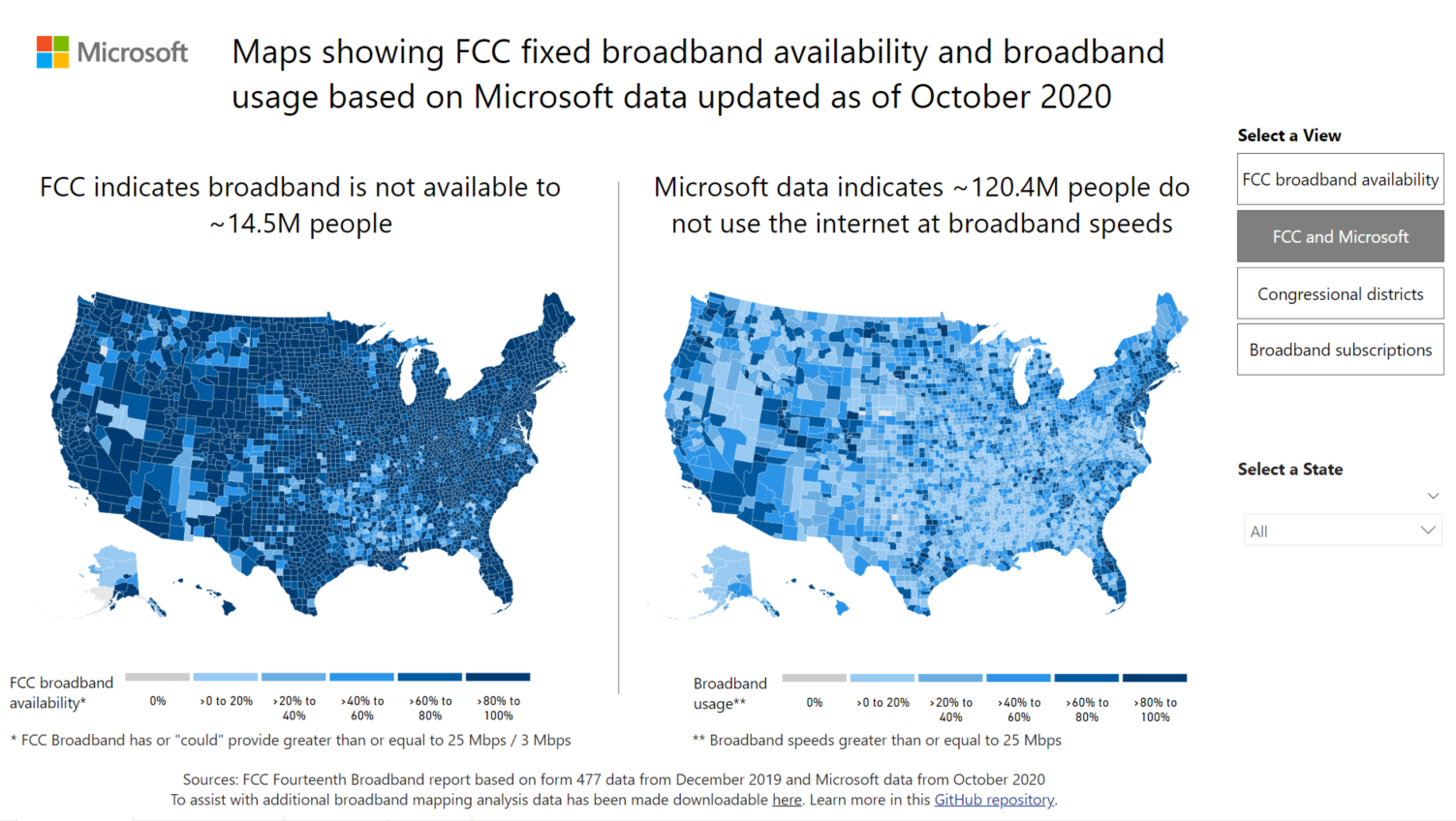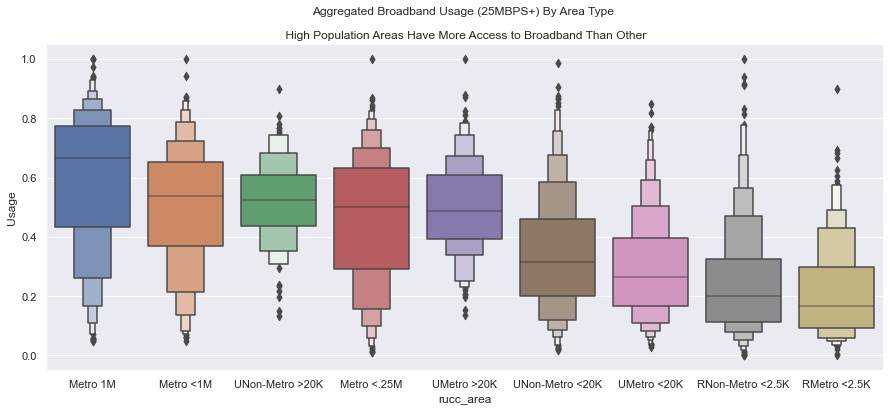## Motivation

I recently came across the Internet Equity Initiative by the Data Science Institute at University of Chicago. They have been studying how disparity in access to internet affects communities across Chicago. Since the pandemic started, we have been working and studying from home. It is critical to have access to not just internet but high-speed broadband internet so families can succeed in this new era of remote work/education. Technology can be a great equalizer but if any disparities exist, it can affect certain communities and put them at a disdvatnage. Microsoft's AI For Good team has been championing the issue of digital equity for a while. They relesed a study and dataset in 2019 that showed that 163M americans lack access to broadband internet, which was significanly higher than FCC's estimate of 25M.I was curious to find out myself how much inequality, if any, exists not just around me but also across the US.In their analysis Microsoft looked at zip code level data. I was curious about two issues:

2. Does median income of a community matter ?

Note here I am interested in access to the internet. A household may have means to purchase high speed internet but that does not mean they have high-speed internet in their area. So I am interested at the aggregate level, how much disparity exists.

## Data

I will be using the data provided by Microsoft's AI For Good team under its Open Data initiative. This was a privatized data to protect privacy. You can read more about the data collection and methods used here. This dataset has zip code, county id and broadband usage percentage. Margin of error is also available but I will disregard that for initial analysis. The usage number shows how many households in that zip code had access to high speed internet (25MBPS+). I will combine this data with the US census data from 2020 to understand the distribution of usage across different areas in US.

```import pandas as pd
import numpy as np
import matplotlib.pyplot as plt
import seaborn as sns
import altair as alt
import statsmodels.api as sm
import statsmodels.formula.api as smf
from IPython.display import IFrame
```

```broadband_data_path = 'https://raw.githubusercontent.com/microsoft/USBroadbandUsagePercentages/master/dataset/broadband_data_zipcode.csv'

def clean_bbdf(path):
'''
Import data and clean column names

'''

df.columns = df.columns.str.replace(" ","_")

return df.set_index('COUNTY_ID')

```
ST COUNTY_NAME POSTAL_CODE BROADBAND_USAGE ERROR_RANGE_(MAE)(+/-) ERROR_RANGE_(95%)(+/-) MSD
COUNTY_ID
45001 SC Abbeville 29639 0.948 0.034 0.110 0.002
45001 SC Abbeville 29620 0.398 0.002 0.007 0.000
45001 SC Abbeville 29659 0.206 0.152 0.608 0.043

#### Check Data Quality

```def data_quality(df):
'''
Check nulls and duplicates

'''
shape = df.shape
is_na = df.isna().sum().any().sum()
is_dupl = df['POSTAL_CODE'].duplicated().any().sum()

return print(f'\n Shape: {shape} ,\n Has nulls: {is_na},\n Has duplicates: {is_dupl}')

data_quality(bbdf)
```
``` Shape: (32735, 7) ,
Has nulls: 0,
Has duplicates: 1
```
```print(f'Number of states: {bbdf.ST.nunique()},  ')
print(f'Number of Counties: {bbdf.index.nunique()}')
```
```Number of states: 51,
Number of Counties: 3136
```
##### Number of Households

The broadband data shows percentage of households with access to the broadband internet so the analysis has to be performed at the household level instead of population. I will use data from US Census to get the numbers.

```#### Household Size

hh_path = 'https://raw.githubusercontent.com/pawarbi/datasets/master/households_2020.csv'

households = (pd.read_csv(hh_path, usecols = ['Geographic Area Name','Estimate!!Total!!HOUSEHOLDS!!Total households'])
.assign(zipcode =  lambda s:s.iloc[:,0].str[5:])
.dropna()
)

households = (households[households['Geographic Area Name'] !="United States"]
.drop('Geographic Area Name', axis=1)
.astype('int')
.set_index('zipcode')
.rename(columns={'Estimate!!Total!!HOUSEHOLDS!!Total households':'households'})

)

```
households
zipcode
601 5555
602 12901
603 19431

Next I will aggregate the data to get weighted usage for each county in the US.

```bbdf_aggregate_usage =  (bbdf.reset_index().set_index('POSTAL_CODE')
.join(households)
.assign(household_usage = lambda s:s['BROADBAND_USAGE'] * s['households'])
.drop(['ERROR_RANGE_(MAE)(+/-)','ERROR_RANGE_(95%)(+/-)','MSD'], axis=1)
.groupby('COUNTY_ID')
.agg(
Combined_Usage = ('household_usage','sum'),
Total_Households = ('households', 'sum')
)
)
```
Combined_Usage Total_Households
COUNTY_ID
1001 8380.027 21450
1003 38315.916 83928
1005 2702.638 8842
```bbdf_aggregate_usage['Usage'] = (np.divide(
bbdf_aggregate_usage['Combined_Usage'].values,
bbdf_aggregate_usage['Total_Households'].values )
)
```
```<ipython-input-62-cc14069b83c1>:1: RuntimeWarning: invalid value encountered in true_divide
bbdf_aggregate_usage['Usage'] = (np.divide(
```
```bbdf_aggregate_usage.head()
```
Combined_Usage Total_Households Usage
COUNTY_ID
1001 8380.027 21450 0.390677
1003 38315.916 83928 0.456533
1005 2702.638 8842 0.305659
1007 846.087 7307 0.115791
1009 3253.546 16802 0.193640

Above is the aggregated broadband usage normalized by households. Next we need to differentiate each county whether it is rural, metro or urban.

#### Rural-urban_Continuum_Code_2003

Rural-Urban Continuum Codes form a classification scheme that distinguishes metropolitan (metro) counties by the population size of their metro area, and nonmetropolitan (nonmetro) counties by degree of urbanization and adjacency to a metro area or areas. For more information from the USDA or to download the original data, go to their section on Rural-Urban Continuum Codes.

##### Rural-Urban Continuum Codes Classification:
• Metro counties:

1 (Counties in metro areas of 1 million population or more)

2 (Counties in metro areas of 250,000 to 1 million population)

3 (Counties in metro areas of fewer than 250,000 population)

• Non-metro counties:

4 (Urban population of 20,000 or more, adjacent to a metro area)

5 (Urban population of 20,000 or more, not adjacent to a metro area)

6 (Urban population of 2,500 to 19,999, adjacent to a metro area)

7 (Urban population of 2,500 to 19,999, not adjacent to a metro area)

8 (Completely rural or less than 2,500 urban population, adjacent to a metro area)

9 (Completely rural or less than 2,500 urban population, not adjacent to a metro area)

88 (Unknown-Alaska/Hawaii State/not official USDA Rural-Urban Continuum code)

99 (Unknown/not official USDA Rural-Urban Continuum code)

```rucc_map = {

1:'Metro 1M',
2:'Metro <1M',
3:'Metro <.25M',
4:'UMetro >20K',
5:'UNon-Metro >20K',
6:'UMetro <20K',
7:'UNon-Metro <20K',
8:'RMetro <2.5K',
9:'RNon-Metro <2.5K',
88: 'UNKNWN',
99:'UNKNWN'
}

county_category = {

1:'Metro',
2:'Metro',
3:'Metro',
4:'Urban',
5:'Urban',
6:'Urban',
7:'Urban',
8:'Rural',
9:'Rural',
88: 'UNKNWN',
99:'UNKNWN'
}
```
```rucc = (pov
.loc[:,['FIPS_code','Stabr','Area_name','Rural-urban_Continuum_Code_2003','MEDHHINC_2020']]
.dropna()
.rename(columns = {'Rural-urban_Continuum_Code_2003':'rucc_codes', 'MEDHHINC_2020':'Median_income'})
.assign(rucc_codes = lambda s:s['rucc_codes'].astype('int'))
.assign(rucc_area = lambda  s:s['rucc_codes'].map(rucc_map))
.assign(county_category = lambda  s:s['rucc_codes'].map(county_category))
.set_index('FIPS_code')

)
```
Stabr Area_name rucc_codes Median_income rucc_area county_category
FIPS_code
1001 AL Autauga County 2 67565.0 Metro <1M Metro
1003 AL Baldwin County 4 71135.0 UMetro >20K Urban
1005 AL Barbour County 6 38866.0 UMetro <20K Urban
```bbdf_county_pov_usage = bbdf_aggregate_usage.join(rucc)

```
Combined_Usage Total_Households Usage Stabr Area_name rucc_codes Median_income rucc_area county_category
COUNTY_ID
1001 8380.027 21450 0.390677 AL Autauga County 2.0 67565.0 Metro <1M Metro
1003 38315.916 83928 0.456533 AL Baldwin County 4.0 71135.0 UMetro >20K Urban
1005 2702.638 8842 0.305659 AL Barbour County 6.0 38866.0 UMetro <20K Urban

#### Distribution of Usage Across Area Types

```sns.set(rc={"figure.figsize":(15, 6)})
median_usage = (bbdf_county_pov_usage
.groupby('rucc_area')['Usage']
.median()
.sort_values(ascending=False)
.round(2)
)
(sns.boxenplot(
data = bbdf_county_pov_usage,
y='Usage', x = 'rucc_area' ,
order = median_usage.index ,
);
``````(sns.kdeplot(
data = bbdf_county_pov_usage,
x='Usage',
hue = 'rucc_area',
palette= {'Metro <1M' : '#fc8d59',
'Metro 1M':'#fc8d59',
'Metro <.25M':'#fc8d59',
'RMetro <2.5K':'blue',
'RNon-Metro <2.5K':'blue',
'UMetro <20K':'#d8daeb',
'UMetro >20K':'#d8daeb',
'UNon-Metro >20K':'#d8daeb',
'UNon-Metro <20K':'#d8daeb'},
fill = True
);

plt.axvline(x=bbdf_county_pov_usage.Usage.median(), label='Median Usage', lw=4, color = 'gray');
plt.text(x = bbdf_county_pov_usage.Usage.median() + 0.025, y = 0.45, s="Median Usage", color='#404040', size='large');
plt.text(x = 0.75, y = 0.3, s="Metro", color='#fc8d59', size='medium');
plt.text(x = -.05, y = 0.3, s="Rural", color='blue', size='medium');
plt.text(x = 0.05, y = 0.45, s="Urban", color='#d8daeb', size='medium');
``````bbdf_county_pov_usage.groupby('rucc_area')['Usage'].median().round(2)
```
```rucc_area
Metro 1M            0.67
Metro <.25M         0.50
Metro <1M           0.54
RMetro <2.5K        0.17
RNon-Metro <2.5K    0.20
UMetro <20K         0.27
UMetro >20K         0.49
UNon-Metro <20K     0.32
UNon-Metro >20K     0.52
Name: Usage, dtype: float64```
```bbdf_county_pov_usage.groupby('county_category')['Usage'].median().round(2)
```
```county_category
Metro    0.56
Rural    0.19
Urban    0.35
Name: Usage, dtype: float64```

### Observations:

Above chart shows distribution of %usage by area types based on RUCC.

1. We can clearly see that rural areas in the US have significantly lower access to broadband internet compared to metro areas.

2. There is ~37% differrence between households living in rural vs metro areas.

3. Metro area shows bi-modal distribution. It's possible certain metro areas have much lower access to broadband internet. I will explore that in future analysis.

4. 67% of households in metros with 1 million or more population have access to broadband !

This is a significant disparity !

```usagev_vs_hh = (bbdf_county_pov_usage.groupby('rucc_area')
.agg(
Median_Usage = ('Usage','median'),
Total_Households = ('Total_Households','sum')
)
)
usagev_vs_hh
```
Median_Usage Total_Households
rucc_area
Metro 1M 0.667304 65090082
Metro <.25M 0.500584 12366652
Metro <1M 0.539444 24873710
RMetro <2.5K 0.168310 958576
RNon-Metro <2.5K 0.200098 1068921
UMetro <20K 0.265684 6111594
UMetro >20K 0.486277 6187898
UNon-Metro <20K 0.316405 3465090
UNon-Metro >20K 0.524717 2360686

### Does Median Income Of the Area Matter?

I combined the median income from US census 2020 with above data to understand the influence of median income.

```sns.set_style("white")
sns.set(rc={"figure.figsize":(10, 6)})
(sns.scatterplot(data= bbdf_county_pov_usage,
x='Median_income',
y ='Usage',
hue = 'county_category',
palette= {'Metro <1M' : '#fc8d59',
'Metro':'#fc8d59',
'Rural':'blue',
'Urban':'#67a9cf',

},alpha=0.7)
plt.show()
``````sns.set(rc={"figure.figsize":(10, 6)})
(sns.lmplot(data= bbdf_county_pov_usage,
x='Median_income',
y ='Usage',
hue = 'county_category',
scatter=False)

``````(sns.kdeplot(data=bbdf_county_pov_usage,
x=(bbdf_county_pov_usage["Median_income"]),
y="Usage",
hue="county_category",
fill=True,
alpha=0.80,
thresh = 0.50,
levels = 10,
palette= {    'Metro':'#fc8d59',
'Rural':'blue',
'Urban':'#67a9cf',

})

```### Observations

Above three plots show relationship between distribution of %households with access to internet vs. the median income.

1. The plots show that there is a moderate positive relationship between the income and %availability. Irrespective of where you live, communities with higher median income have higher chance to having access to the broadband internet

2. Notice the slope of the lines in the linear fit plot. Metro line has a slightly more slope compared to rural. This shows that income inequality affects metro areas more than the rural areas.

3. In rural areas, even higher income communities cannot get access to high speed internet. I think this points to the discrepancy in the availability and infrastructure. Rural communities in US do not yet have access to the high speed internet.

4. Another pointer towards the infrastructure issue is the differrence between the intercepts. For example, notice that even for lower income communities in metro areas, roughly 30% have access to broadband internet whereas in rural areas, it's almost non-existent.

5. The density plot above shows 50% of the density for each of the areas. The separation between them clearly shows the discrepancy between rural and metro areas.

##### Quantile Regression

Next I wanted to understand how different income communities are affected. This can be done with quantile regression where I fit linear regression to 10%, 25%, 50%, 75% and 90% quantile of usage.

```qdata = bbdf_county_pov_usage.drop(['Combined_Usage','Total_Households','rucc_codes'],axis=1).dropna()
qmodel = smf.quantreg('Usage~Median_income',qdata)

qs = [10,25,50,75,90]

for q in qs:
res = qmodel.fit(q/100)
#     qdata["Q"+str(q)]=[(res.params + x*res.params) for x in qdata.Median_income]

qdata["Q"+str(q)]=qmodel.predict(res.params)

```
Usage Stabr Area_name Median_income rucc_area county_category Q10 Q25 Q50 Q75 Q90
COUNTY_ID
1001 0.390677 AL Autauga County 67565.0 Metro <1M Metro 0.197055 0.335295 0.481372 0.621848 0.725269
1003 0.456533 AL Baldwin County 71135.0 UMetro >20K Urban 0.215659 0.366749 0.517384 0.655024 0.752876
1005 0.305659 AL Barbour County 38866.0 UMetro <20K Urban 0.047497 0.082435 0.191876 0.355154 0.503341
1007 0.115791 AL Bibb County 50907.0 Metro 1M Metro 0.110246 0.188525 0.313338 0.467049 0.596454
1009 0.193640 AL Blount County 55203.0 Metro 1M Metro 0.132633 0.226376 0.356673 0.506971 0.629674
```qmodel = smf.quantreg('Usage~Median_income',qdata)

qs = [10,25,50,75,90]

for q in qs:
res = qmodel.fit(q/100)
#     qdata["Q"+str(q)]=[(res.params + x*res.params) for x in qdata.Median_income]

qdata["Q"+str(q)]=qmodel.predict(res.params)
```
```(alt.Chart(qdata).mark_circle(opacity=0.4).encode(
x = 'Median_income:Q',
y = 'Usage:Q',
color=alt.Color('county_category', scale=alt.Scale(scheme='set2', range=['#fc8d59','blue','#67a9cf',])),
tooltip = ['county_category']
)) + (
alt.Chart(qdata).mark_line(opacity = 0.6, color='black').encode(
x = 'Median_income:Q',
y = 'Q10:Q'
)) + (
alt.Chart(qdata).mark_line(opacity = 0.6, color='gray', size=1.5).encode(
x = 'Median_income:Q',
y = 'Q25:Q'

)) + (alt.Chart(qdata).mark_line(opacity = 0.6, color='gray', size=1.5).encode(
x = 'Median_income:Q',
y = 'Q50:Q')) + (
alt.Chart(qdata).mark_line(opacity = 0.6, color='gray', size=1.5).encode(
x = 'Median_income:Q',
y = 'Q75:Q')) + (
alt.Chart(qdata).mark_line(opacity = 0.6, color='black',).encode(
x = 'Median_income:Q',
y = 'Q90:Q')
).interactive().properties(width = 600, height = 600,
title = {
"text": ["Broadband Usage vs. Median Income", "By County Area Type"],
"subtitle": ["\n Black lines show quantile regression predictions @ 10% and 90%", "\n\nHouseholds with higher median income have grater access to broadband internet compared to lower income housholds"],
"color": "black",
"subtitleColor": "black",

}) + (alt.Chart({'values':[{'x': 160000, 'y': 0.7}]}).mark_text(
text='10th Quantile', angle=0).encode(
x='x:Q', y='y:Q'
)) + (alt.Chart({'values':[{'x': 160000, 'y': 1.45}]}).mark_text(
text='90th Quantile', angle=0).encode(
x='x:Q', y='y:Q'
))
```